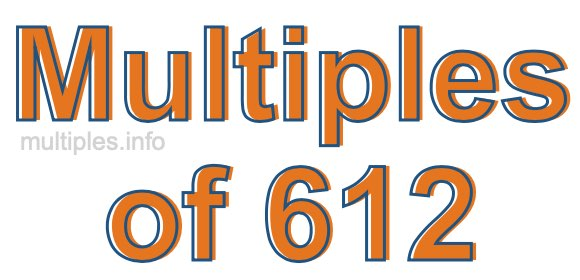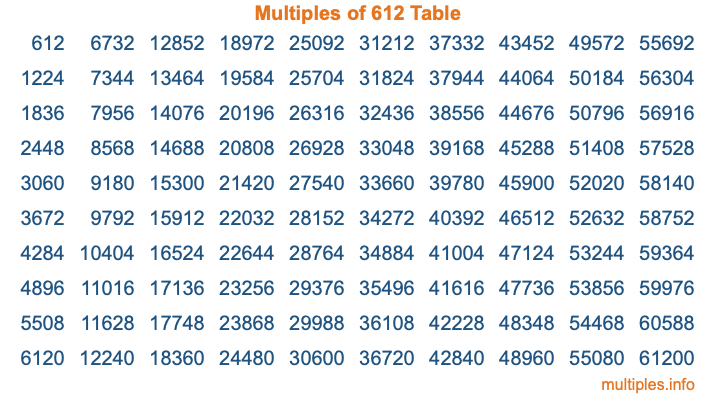Multiples of 612Welcome to the Multiples of 612 page. Here we will first teach you everything you will ever need to know about the multiples of 612, and then give you a study guide summary of everything we taught you to make sure you remember it all. Use this page to look up facts and learn information about the multiples of 612. This page will make you a multiples of six hundred twelve expert!

Definition of Multiples of 612
Multiples of 612 are all the numbers that when divided by 612 equal an integer. Each of the multiples of 612 are called a multiple. A multiple of 612 is created by multiplying 612 by an integer.

Therefore, to create a list of multiples of 612, you start with 1 multiplied by 612, then 2 multiplied by 612, then 3 multiplied by 612, and so on for as long as you want. Thus, the list of the first five multiples of 612 is 612, 1224, 1836, 2448, and 3060. To see a larger list of multiples of 612, see the printable image of Multiples of 612 further down on this page. We also have a category where you can choose any nth multiple of 612.

Multiples of 612 Checker
The Multiples of 612 Checker below checks to see if any number of your choice is a multiple of 612. In other words, it checks to see if there is any number (integer) that when multiplied by 612 will equal your number. To do that, we divide your number by 612. If the the quotient is an integer, then your number is a multiple of 612.

Is  a multiple of 612?

Least Common Multiple of 612 and ...
A Least Common Multiple (LCM) is the lowest multiple that two or more numbers have in common. This is also called the smallest common multiple or lowest common multiple and is useful to know when you are adding our subtracting fractions. Enter one or more numbers below (612 is already entered) to find the LCM.

Check out our LCM Calculator if you need more details about the Least Common Multiple or if you need the LCM for different numbers for adding and subtraction fractions.

nth Multiple of 612
As we stated above, 612 is the first multiple of 612, 1224 is the second multiple of 612, 1836 is the third multiple of 612, and so on. Enter a number below to find the nth multiple of 612.

th multiple of 612

Multiples of 612 vs Factors of 612
612 is a multiple of 612 and a factor of 612, but that is where the similarities end. All postive multiples of 612 are 612 or greater than 612. All positive factors of 612 are 612 or less than 612.

Below is the beginning list of multiples of 612 and the factors of 612 so you can compare:

Multiples of 612: 612, 1224, 1836, 2448, 3060, etc.

Factors of 612: 1, 2, 3, 4, 6, 9, 12, 17, 18, 34, 36, 51, 68, 102, 153, 204, 306, 612

As you can see, the multiples of 612 are all the numbers that you can divide by 612 to get a whole number. The factors of 612, on the other hand, are all the whole numbers that you can multiply by another whole number to get 612.

It's also interesting to note that if a number (x) is a factor of 612, then 612 will also be a multiple of that number (x).

Multiples of 612 vs Divisors of 612
The divisors of 612 are all the integers that 612 can be divided by evenly. Below is a list of the divisors of 612.

Divisors of 612: 1, 2, 3, 4, 6, 9, 12, 17, 18, 34, 36, 51, 68, 102, 153, 204, 306, 612

The interesting thing to note here is that if you take any multiple of 612 and divide it by a divisor of 612, you will see that the quotient is an integer.

Multiples of 612 Table
Below is an image of the first 100 multiples of 612 in a table. The table is in chronological order, column by column. The first column has the first ten multiples of 612, the second column has the next ten multiples of 612, and so on.The Multiples of 612 Table is also referred to as the 612 Times Table or Times Table of 612. You are welcome to print out our table for your studies.

Negative Multiples of 612
Although not often discussed or needed in math, it is worth mentioning that you can make a list of negative multiples of 612 by multiplying 612 by -1, then by -2, then by -3, and so on, to get the following list of negative multiples of 612:

-612, -1224, -1836, -2448, -3060, etc.

Multiples of 612 Summary
Below is a summary of important Multiples of 612 facts that we have discussed on this page. To retain the knowledge on this page, we recommend that you read through the summary and explain to yourself or a study partner why they hold true.

There are an infinite number of multiples of 612.

A multiple of 612 divided by 612 will equal a whole number.

612 divided by a factor of 612 equals a divisor of 612.

The nth multiple of 612 is n times 612.

The largest factor of 612 is equal to the first positive multiple of 612.

612 is a multiple of every factor of 612.

612 is a multiple of 612.

A multiple of 612 divided by a divisor of 612 equals an integer.

612 divided by a divisor of 612 equals a factor of 612.

Any integer times 612 will equal a multiple of 612.

Multiples of a Number
Here you can get the multiples of another number, all with the same attention to detail as we did for multiples of 612 on this page.

Multiples of
Multiples of 613
Did you find our page about multiples of six hundred twelve educational? Do you want more knowledge? Check out the multiples of the next number on our list!